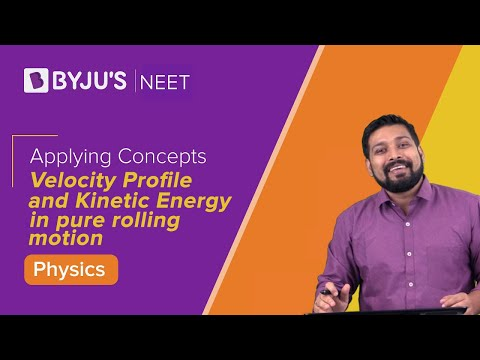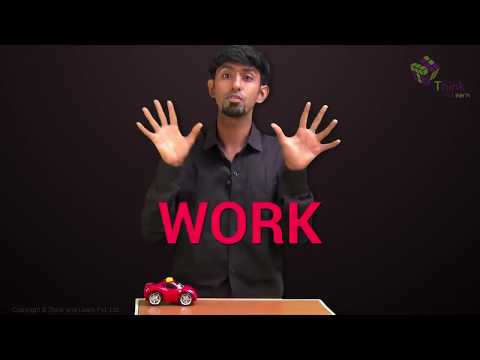# Work, Energy and Power MCQ for NEET

Work, energy, and power are the most commonly used terms in Physics.

Work is defined as the transfer of energy. In Physics, work is said to be done when there is a transfer of energy from one body to another. Also, work is an application of force.

Energy is defined as the capacity to do work. Kinetic energy and potential energy are the two types of energy. While heat energy, mechanical energy, chemical energy are the forms of energy.

Power is defined as the work done in unit time.

1. By how much does kinetic energy increase if the momentum is increased by 20%

(a) 55%

(b) 66%

(c) 44%

(d) 77%

2. Calculate the energy loss in a perfectly inelastic collision if the mass of the object is 40kg with velocity 4m/s hits the object of mass 60kg with velocity 2m/s.

(a) 440J

(b) 110J

(c) 392J

(d) 48J

3. Calculate the final kinetic energy of a block of mass if the mass of the block is 10kg and has a constant velocity of 10m/s. The block of mass is subjected to a retarding force of F=0.1J/m

(a) 275J

(b) 250J

(c) 475J

(d) 450J

4. What is the average power required to lift a mass of 100kg to a height of 50m in 50 seconds?

(a) 980

(b) 100

(c) 50

(d) 5000

5. What is the power of the engine when the velocity of the car is v, mass m, acceleration a, and external resistance R

(a) (R-ma)v

(b) (R+ma)v

(c) mav

(d)Rv

6. Consider an object with m as its mass such that it is accelerated uniformly from rest and the speed attained by the object is v in T time.  Calculate the instantaneous power that is delivered to the body in terms of function of time

(a) $\frac{1}{2}\frac{mv^{2}}{T^{2}}t$

(b) $\frac{1}{2}\frac{mv^{2}}{T^{2}}t^{2}$

(c) $\frac{mv^{2}}{T^{2}}t$

(d) $\frac{mv^{2}}{T^{2}}t^{2}$

Answer: (b) $\frac{1}{2}\frac{mv^{2}}{T^{2}}t^{2}$

7. A ball is thrown from a height of 20 m vertically downward and has an initial velocity of $v_{0}^{2}$. As the stone collides with the ground 50% of its energy is lost and bounce back to the same height. What is the initial velocity?

(a) 28m/s

(b) 20m/s

(c) 10m/s

(d) 14m/s

8. Consider a particle of mass m which is driven by a machine that has a constant power k Watts. What is the force on the particle at time t if the particle starts from the rest?

(a) $\sqrt{2mk}\;t^{\frac{-1}{2}}$

(b) $\frac{1}{2}\sqrt{mk}\;t^{\frac{-1}{2}}$

(c) $\sqrt{mk}\;t^{\frac{-1}{2}}$

(d) $\sqrt{\frac{mk}{2}}t^{\frac{-1}{2}}$

Answer: (d) $\sqrt{\frac{mk}{2}}t^{\frac{-1}{2}}$

9. When can one say that work is done on the body

(a) When the body experiences force

(b) When there is an increase in energy because of mechanical influence

(c) When the body moves a certain distance

(d) None of the above

Answer: (b) When there is an increase in energy because of mechanical influence

10. Select the incorrect statement for the given situation: Two bodies with mass m1 and m2 undergo elastic collision

(a) When m1 and m2 is at rest, the kinetic energy transferred will be maximum

(b) The bodies will move in the same direction if m1 = m2 and when the collision is in oblique

(c) When m1 > m2, m2 will move with four times increase in its velocity than m1

(d) When m1 < m2, the transfer of momentum will be maximum

Answer: (c) When m1 > m2, m2 will move with four times increase in its velocity than m1

12. What is the ratio of velocities of a nucleus that splits into two parts with radii 1:2

(a) 6:1

(b) 2:1

(c) 8:1

(d) 4:1

13. What is the relation between E, P, and E if E is kinetic energy, P is momentum, and V is the velocity of the particle

(a) $P=\frac{dV}{dt}$

(b) $P=\frac{dE}{dt}$

(c) $P=\frac{dE}{dV}\times \frac{dE}{dt}$

(d) $P=\frac{dE}{dV}$

Answer: (d) $P=\frac{dE}{dV}$Recommended NEET Physics MCQs:

### Recommended Video on Work, Energy and Power:1. Good